### OUTLINE OF ELEMENTARY AND INTERMEDIATE ALGEBRA

Important Definitions
Factors; coefficient; exponent; power; base; term; algebraic sum; similar terms; degree; homogeneous expression; linear equation; root of an equation; root of an expression; identity; conditional equation; prime quantity; highest common factor (H. C. F.); lowest common multiple (L. C. M.); involution; evolution; imaginary number; real number; rational; similar radicals; binomial surd; pure quadratic equation; affected quadratic equation; equation in the quadratic form; simultaneous linear equations; simultaneous quadratic equations; discriminant; symmetrical expression; ratio; proportion; fourth proportional; third proportional; mean proportional; arithmetic progression; geometric progression;Special Rules for Multiplication and Division
1. Square of the sum of two quantities.2. Square of the difference of two quantities.3. Product of the sum and difference of two quantities.4. Product of two binomials having a common term.5. Product of two binomials whose corresponding terms are similar.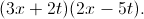6. Square of a polynomial.7. Sum of two cubes.8. Difference of two cubes.9. Sum or difference of two like powers.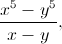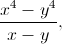Cases in Factoring
1. Common monomial factor.2. Trinomial that is a perfect square.3. The difference of two squares.
(a) Two terms.(b) Four terms.(c) Six terms.(d) Incomplete square.4. Trinomial of the form5. Trinomial of the form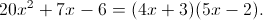6. Sum or difference of
• two cubes. See "Special Rules," 7 and 8.
• two like powers. See "Special Rules," 9.
7. Common polynomial factor. Grouping.8. Factor Theorem.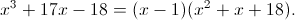H. C. F. and L. C. M.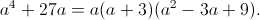H. C. F.L. C. M.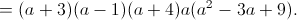Fractions
Reduction to lowest terms.
Reduction of a mixed number to an improper fraction.
Reduction of an improper fraction to a mixed number.
Multiplication and division of fractions.
Law of signs in division, changing signs of factors, etc.
Complex fractions.
Simultaneous Equations
Solved by
• substitution.
• comparison.
Graphical representation.
Involution
Law of signs.
Binomial theorem laws.
Expansion of
• monomials and fractions.
• binomials.
• trinomials.
Evolution
Law of signs.
Evolution of monomials and fractions.
Square root of algebraic expressions.
Square root of arithmetical numbers.
Optional
• Cube root of algebraic expressions.
• Cube root of arithmetical numbers.
Theory of Exponents
Proofs: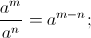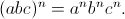Meaning of
• fractional exponent.
• zero exponent.
• negative exponent.
Four rules
• To multiply quantities having the same base, add exponents.
• To divide quantities having the same base, subtract exponents.
• To raise to a power, multiply exponents.
• To extract a root, divide the exponent of the power by the index of the root.
• Fraction under the radical sign.
• Reduction to an entire surd.
• Changing to surds of different order.
• Reduction to simplest form.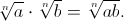Rationalization
• Monomial denominator.
• Binomial denominator.
• Trinomial denominator.
Square root of a binomial surd.
Radical equations. Always check results to avoid extraneous roots.
Pure.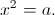Affected.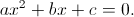Methods of solving
• Completing the square.
• Formula. Developed from• Factoring.
•• Then• Discriminant,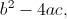and its discussion.
• Nature or character of the roots.
Case I.
One equation linear.Case II.
Both equations homogeneous and of the second degree.Case III.
Any two of the quantities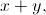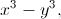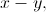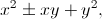etc., given.Case IV.
Both equations symmetrical or symmetrical except for sign. Usually one equation of high degree, the other of the first degree.Case V. Special Devices
I. Solve for a compound unknown, likeetc., first.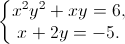II. Divide the equations, member by member.Ratio and Proportion
Proportionals
• mean,
• third,
• fourth.
Theorems
1. Product of means equals product of extremes.
2. If the product of two numbers equals the product of two other numbers, either pair, etc.
3. Alternation.
4. Inversion.
5. Composition.
6. Division.
7. Composition and division.
8. In a series of equal ratios, the sum of the antecedents is to the sum of the consequents as any antecedent, etc.
Special method of proving four quantities in proportion. Let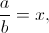etc.
Progressions
Development of formulas.Insertion of means
• Arithmetical.
• Geometrical.
Binomial Theorem
Review of binomial theorem laws. See Involution.
Expansion ofFinding any term by
• key number method.
•orterm method.